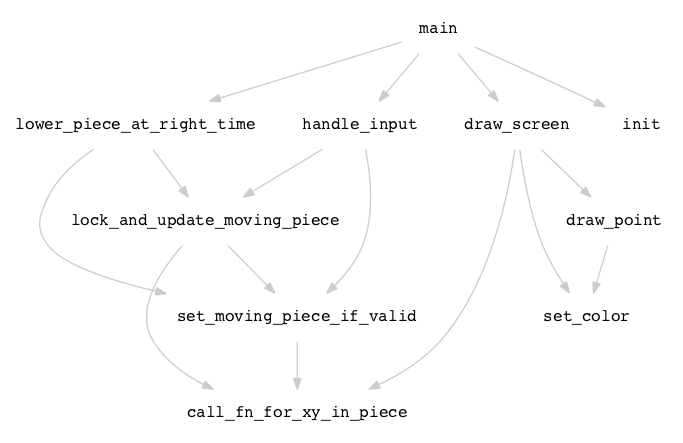Skip to content
{{ message }}

Instantly share code, notes, and snippets.

# tylerneylon/call_graph.awk

Created Oct 7, 2014
Parse Lua code and draw its call graph.
 #!/usr/bin/awk -f # # call_graph.awk # # Usage: # ./call_graph.awk my_program.lua | dot -Tpng > call_graph.png # # This is a script that generates a visual call graph # for a Lua file. This script only shows calls made # to functions defined within the input Lua file; that is, # it excludes calls such as standard library or built-in functions. # # Use the option "-v nodirect=1" to hide direct function calls; # these are calls made from the top level of your Lua program. # Example: ./call_graph.awk -v nodirect=1 my_program.lua # BEGIN { identifier = "[A-Za-z_][A-Za-z0-9_]*" fn_call = identifier "\\\(" in_fn = "\"\"" if (!nodirect) defined_fns[in_fn] = 1 print "strict digraph {" print " node [shape=box color=\"#FFFFFF\" fontname=\"courier\" fontsize=12];" print " edge [color=\"#CCCCCC\" arrowsize=0.8];" } # Detect and ignore comments. /^--\[\[/ { in_a_comment = 1 } /^--\]\]/ { in_a_comment = 0 } # Detect when we are at the top-level of the program; out of any functions. # This detection is not foolproof; it assumes the code is consistently indented. /^ *end/ { indent = match(\$0, /[^ ]/) - 1 if (indent == in_fn_indent) { in_fn = "\"\"" } } # Detect and track function definitions. # This includes only functions declared at the start of a line. /^ *(local *)?function/ { if (in_a_comment) next if (\$1 == "local") { fn_name = \$3 } else { fn_name = \$2 } sub(/\(.*/, "", fn_name) defined_fns[fn_name] = 1 in_fn = fn_name in_fn_indent = match(\$0, /[^ ]/) - 1 next # Don't consider this line as a function call. } # Track function calls. \$0 ~ fn_call { if (in_a_comment) next fn_index = match(\$0, fn_call) comment_index = match(\$0, /--/) if (comment_index && comment_index < fn_index) next tail = substr(\$0, match(\$0, fn_call)) fn_name = substr(tail, 1, index(tail, "\(") - 1) calls[in_fn " -> " fn_name] = 1 # Uncomment the following line to help debug this script. #printf "%d: called_fns[%s] = %s\n", NR, fn_name, in_fn } END { for (call in calls) { split(call, fns, " -> ") if (defined_fns[fns] && defined_fns[fns]) { print " " call } } print "}" }

###tylerneylon commented Oct 7, 2014

 This is a script to draw a call graph for your Lua code. It runs in `awk` and generates output, on stdout, designed as input to Graphviz via the `dot` command. Here's an example command run on the input file `termtris.lua`: ``````\$ ./call_graph.awk termtris.lua | dot -Tpng > call_graph.png `````` Here's the example image generated by the above command line:###sineer commented Nov 24, 2014

 It doesn't work for me and I can't figure out why... cluster@jHP:~\$ call_graph.awk termtris.lua strict digraph { node [shape=box color="#FFFFFF" fontname="courier" fontsize=12]; edge [color="#CCCCCC" arrowsize=0.8]; awk: run time error: regular expression compile failed (missing ')') [A-Za-z_][A-Za-z0-9_]*( FILENAME="termtris.lua" FNR=1 NR=1

###ClaudeM commented Mar 23, 2015

 Hi, The script did not work with the code we have because it uses functions with doted names, e.g., app.doSomething. Here is the edited version of the script. There are other small changes; some were made to help debugging and others just seemed like a good idea at the time. :-) ``````#!/usr/bin/awk -f # # call_graph.awk # # Usage: # ./call_graph.awk my_program.lua | dot -Tpng > call_graph.png # # This is a script that generates a visual call graph for a Lua file. # This script only shows calls made to functions defined within the # input Lua file; that is, it excludes calls such as standard library # or built-in functions. # # Use the option "-v nodirect=1" to hide direct function calls; these # are calls made from the top level of your Lua program. # # Example: ./call_graph.awk -v nodirect=1 my_program.lua # BEGIN { identifier = "[A-Za-z_][A-Za-z0-9_.]+" fn_call = identifier "\\(" in_fn = "_direct_call_" if (!nodirect) defined_fns[in_fn] = 1 print "strict digraph {" print " node [shape=box color=\"#FFFFFF\" fontname=\"courier\" fontsize=12];" print " edge [color=\"#CCCCCC\" arrowsize=0.8];" } # Detect and ignore comments. /^--\[\[/ { in_a_comment = 1 } /^--\]\]/ { in_a_comment = 0 } # Detect when we are at the top-level of the program; out of any functions. # This detection is not foolproof; it assumes the code is consistently indented. /^ *end/ { indent = match(\$0, /[^ ]/) - 1 if (indent == in_fn_indent) { in_fn = "_direct_call_" } } # Detect and track function definitions. # This includes only functions declared at the start of a line. /^ *(local *)?function/ { if (in_a_comment) next if (\$1 == "local") { fn_name = \$3 } else { fn_name = \$2 } sub(/\(.*/, "", fn_name) defined_fns[fn_name] = 1 in_fn = fn_name in_fn_indent = match(\$0, /[^ ]/) - 1 # Uncomment the following line to help debug this script. # printf "%d: --- start function %s\n", NR, fn_name next # Don't consider this line as a function call. } # Track function calls. \$0 ~ fn_call { if (in_a_comment) next fn_index = match(\$0, fn_call) comment_index = match(\$0, /--/) if (comment_index && comment_index < fn_index) next tail = substr(\$0, match(\$0, fn_call)) fn_name = substr(tail, 1, index(tail, "(") - 1) calls[in_fn " -> " fn_name] = 1 # Uncomment the following line to help debug this script. # printf "%d: called_fns[%s] = %s\n", NR, fn_name, in_fn } END { for (call in calls) { split(call, fns, " -> ") if (defined_fns[fns] && defined_fns[fns]) { print " \"" fns "\" -> \"" fns "\"" } } print "}" } ``````
to join this conversation on GitHub. Already have an account? Sign in to comment